# Test: Boundary Layer Level - 1

## 10 Questions MCQ Test Engineering Mechanics | Test: Boundary Layer Level - 1

Description
Attempt Test: Boundary Layer Level - 1 | 10 questions in 25 minutes | Mock test for Mechanical Engineering preparation | Free important questions MCQ to study Engineering Mechanics for Mechanical Engineering Exam | Download free PDF with solutions
QUESTION: 1

### The transition Reynolds number for flow over a flat plate is 5 × 105. What is the distance from the leading edge at which transition will occur for flow of water with a free stream velocity of 1 m/s ? (For water, the kinematic viscosity is ν = 0.86 × 10−6 m2/s)

Solution: Rex = Vx / ν

Substituting values

5 × 105 = 1 × x / 0.86 × 10−6

x = 0.43 m

QUESTION: 2

### The laminar boundary layer thickness varies as

Solution: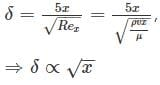QUESTION: 3

### Under what condition does flow separation take place?

Solution: Flow separation can only take place when, there is adverse pressure gradient i.e as the fluid moves forward along the plate (dx > 0) the pressure increases dp > 0 hence for flow separation dp / dx > 0

QUESTION: 4

Friction drag is generally larger than the pressure drag in

Solution: The pressure drag will be maximum in case of flow separations. So in case of flow past a cylinder or sphere, there will be considerable pressure drag as in these cases, the flow separates early on. In case of flow past a thin sheet, there will be no pressure drag (Assumptions of Blasius). In case of air foil, the pressure drag will exist but it will be smaller than friction drag.

QUESTION: 5

A fluid is flowing over a flat plate. At a distance of 8 cm from the leading edge, the Reynolds number is found to be 25600. The thickness of the boundary layer at this point is

Solution: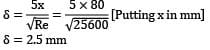QUESTION: 6

In a laminar boundary layer over a flat plate, what would be the ratio of wall shear stresses τ1 and τ2 at two sections which lie at distance x1 = 2 units and x2 = 10 units from the leading edge of the plate?

Solution:

For laminar boundary layer,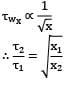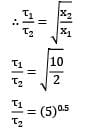Hence option (B) is the answer.

QUESTION: 7

Consider the following statements pertaining to boundary layer on solid surfaces:

1. The boundary layer separation takes place if the pressure gradient is zero.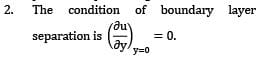Boundary layer on a flat plate is laminar if the Reynolds number is less than 5 × 105.

Which of the above statements is/are correct?

Solution: Boundary layer can only separate if there is an adverse pressure gradient in the flow direction, without a pressure gradient, the boundary layer will never separate, so 1 is wrong. For Boundary layer to separate, the fluid near the boundary will also have to come to rest, along with the fluid particles which are on the surface. Hence velocity gradient at the wall (y = 0) will be 0.

QUESTION: 8

The velocity distribution in a laminar boundary layer over a flat plate has been prescribed by the identity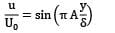The factor A will have an approximate value of

Solution: For laminar boundary layer the velocity profile should definitely satisfy the following boundary conditions.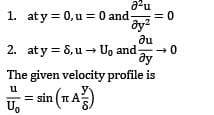t satisfies the first condition as at y = 0, sin term becomes zero. Let us consider the second condition i. e. at y = δ, u → U0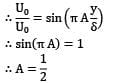Hence option (A) is the answer.

QUESTION: 9

The velocity distribution in the laminar boundary layer is presumed to conform to the identity u /U0 = y / δ . The momentum thickness for this laminar boundary layer would be

Solution: The momentum thickness (θ) for a boundary layer is given as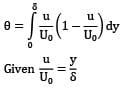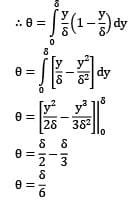Hence option (C) is the answer.

QUESTION: 10

Angle of diverging portion of the venturimeter is limited to 7°, because

1. Flow decelerates in the diverging portion and pressure increases in the downstream direction. Hence, the fluid experience an adverse pressure gradient, if the divergence angle is large.

2. Flow separation takes place due to adverse pressure gradient when divergence angle is large.

3. If the divergence angle is large, a negative pressure is created at the throat which obstructs the flow of fluid.

Which of the above reasons are correct?

Solution: The pressure at the throat is not affected by the divergence angle, it depends on inlet pressure, inlet diameter and throat diameter (Bernoulli’s equation) whereas the flow separation is affected by the divergence angle.Use Code STAYHOME200 and get INR 200 additional OFF Use Coupon Code